# Tutorial Aims:

## Quantifying population change

This workshop will provide an overview of methods used to investigate an ecological research question using a big(ish) dataset that charts the population trends of ~15,000 animal populations from ~3500 species across the world, provided by the Living Planet Index Database. We will use the LPI dataset to first examine how biodiversity has changed since 1970, both globally and at the biome scale, and then we will zoom in further to create a map of the distribution of the Atlantic puffin based on occurrence data from Global Biodiversity Information Facility and Flickr. We will be following a generic workflow that is applicable to most scientific endeavours, at least in the life sciences. This workflow can be summed up in this diagram we recreated from Hadley Wickham’s book R for Data Science:All the resources for this tutorial, including some helpful cheatsheets can be downloaded from this repository Press the green ‘Code’ button and download the repo as a zipfile, then unzip and set the folder as your working directory by running the code below (subbing in the actual folder path), or clicking `Session/ Set Working Directory/ Choose Directory` from the RStudio menu.

Alternatively, you can fork the repository to your own Github account and then add it as a new RStudio project by copying the HTTPS/SSH link. For more details on how to register on Github, download Git, sync RStudio and Github and use version control, please check out our previous tutorial.

Make a new script file using `File/ New File/ R Script` and we are all set to begin exploring how vertebrate populations are changing.

``````setwd("PATH_TO_FOLDER")
``````

Next, install (`install.packages("")`) and load (`library()`) the packages needed for this tutorial.

``````install.packages("readr")
install.packages("tidyr")
install.packages("dplyr")
install.packages("broom")
install.packages("ggplot2")
install.packages("ggExtra")
install.packages("maps")
install.packages("RColorBrewer")

library(tidyr)
library(dplyr)
library(broom)
library(ggplot2)
library(ggExtra)
library(maps)
library(RColorBrewer)
``````

Finally, load the `.RData` files we will be using for the tutorial. The data we originally downloaded from the LPI website were in a `.csv` format, but when handling large datasets, `.RData` files are quicker to use, since they are more compressed. Of course, a drawback would be that `.RData` files can only be used within R, whereas `.csv` files are more transferable.

``````load("LPIdata_Feb2016.RData")
``````

# 1. Formatting and tidying data using `tidyr`

## Reshaping data frames using `gather()`

The way you record information in the field or in the lab is probably very different to the way you want your data entered into R. In the field, you want tables that you can ideally draw up ahead and fill in as you go, and you will be adding notes and all sorts of information in addition to the data you want to analyse. For instance, if you monitor the height of seedlings during a factorial experiment using warming and fertilisation treatments, you might record your data like this: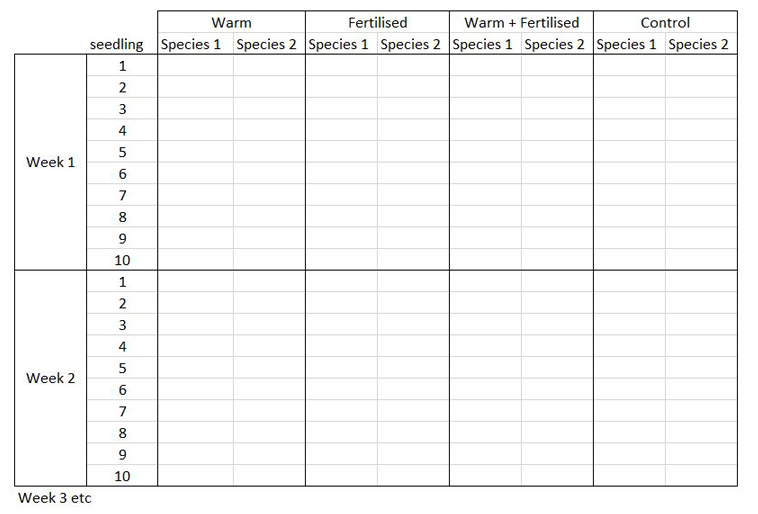Let’s say you want to run a test to determine whether warming and/or fertilisation affected seedling growth. You may know how your experiment is set up, but R doesn’t! At the moment, with 8 measures per row (combination of all treatments and species for one replicate, or block), you cannot run an analysis. On the contrary, tidy datasets are arranged so that each row represents an observation and each column represents a variable. In our case, this would look something like this: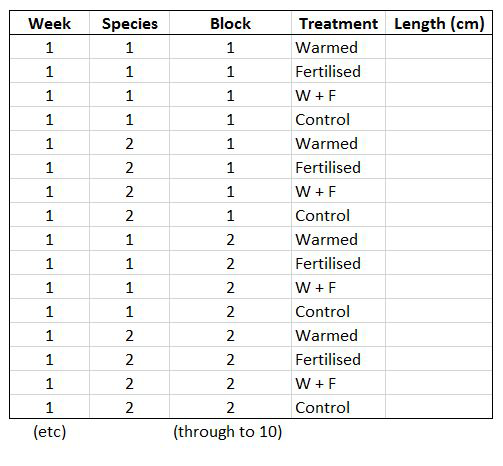This makes a much longer dataframe row-wise, which is why this form is often called long format. Now if you wanted to compare between groups, treatments, species, etc, R would be able to split the dataframe correctly, as each grouping factor has its own column.

The `gather()` function from the `tidyr` package lets you convert a wide-format data frame to a tidy long-format data frame.

Have a look at the first few columns of the LPI data set (`LPIdata_Feb2016`) to see whether it is tidy:

``````View(head(LPIdata_Feb2016))
``````

At the moment, each row contains a population that has been monitored over time and towards the right of the data frame there are lots of columns with population estimates for each year. To make this data “tidy” (one column per variable) we can use `gather()` to transform the data so there is a new column containing all the years for each population and an adjacent column containing all the population estimates for those years:

``````LPI_long <- gather(data = LPIdata_Feb2016, key = "year", value = "pop", 26:70)
``````

This takes our original dataset `LPIdata_Feb2016` and creates a new column called `year`, fills it with column names from columns `26:70` and then uses the data from these columns to make another column called `pop`.

Because column names are coded in as characters, when we turned the column names (`1970`, `1971`, `1972`, etc.) into rows, R automatically put an `X` in front of the numbers to force them to remain characters. We don’t want that, so to turn `year` into a numeric variable, use:

``````LPI_long\$year <- parse_number(LPI_long\$year)
``````

## Using sensible variable names

Have a look at the column names in `LPI_long`:

``````names(LPI_long)
``````

The variable names are a mixture of upper and lower case letters and some use `.` to mark the end of a word. There are lots of conventions for naming objects and variables in programming but for the sake of consistency and making things easier to read, let’s replace any `.` with `_` using `gsub()`and make everything lower case using `tolower()`:

``````names(LPI_long) <- gsub(".", "_", names(LPI_long), fixed = TRUE)
names(LPI_long) <- tolower(names(LPI_long))
``````

Each population in the dataset can be identified using the `id` column, but to group our dataset by species, we would have to group first by `genus`, then by `species`, to prevent problems with species having similar names. To circumvent this, we can make a new column which holds the genus and species together using `paste()`:

``````LPI_long\$genus_species_id <- paste(LPI_long\$genus, LPI_long\$species, LPI_long\$id, sep = "_")
``````

Finally, lets look at a sample of the contents of the data frame to make sure all the variables are displayed properly:

``````View(LPI_long[c(1:5,500:505,1000:1005),])
# You can use [] to subset data frames [rows, columns]
# If you want all rows/columns, add a comma in the row/column location
``````

`country_list` and `biome` seem to have `,` and `/` to separate entries. This could mess up our analyses, so we can remove them using `gsub()` as before:

``````LPI_long\$country_list <- gsub(",", "", LPI_long\$country_list, fixed = TRUE)
LPI_long\$biome <- gsub("/", "", LPI_long\$biome, fixed = TRUE)
``````

# 2. Efficiently manipulating data using `dplyr`

Now that our dataset is tidy we can get it ready for our analysis. This data frame contains data from lots of different sources so to help answer our question of how populations have changed since 1970, we should create some new variables and filter out the unnecessary data.

To make sure there are no duplicate rows, we can use `distinct()`:

``````LPI_long <- distinct(LPI_long)
``````

Then we can remove any rows that have missing or infinite data:

``````LPI_long_fl <- filter(LPI_long, is.finite(pop))
``````

Next, we want to only use populations that have more than 5 years of data to make sure our analysis has enough data to capture population change. We should also scale the population data, because since the data come from many species, the units and magnitude of the data are very different - imagine tiny fish whose abundance is in the millions, and large carnivores whose abundance is much smaller. Scaling also normalises the data, as later on we will be using linear models assuming a normal distribution. To only keep populations with more than 5 years of data and scale the population data, we can use pipes.

Pipes (`%>%`) are a way of streamlining data manipulation - imagine all of your data coming in one end of the pipe, while they are in there, they are manipulated, summarised, etc., then the output (e.g. your new data frame or summary statistics) comes out the other end of the pipe. At each step of the pipe processing, you can tell the pipe what information to use - e.g. here we are using `.`, which just means “take the ouput of the previous step”. For more information on data manipulation using pipes, you can check out our data formatting and manipulation tutorial.

``````LPI_long <- LPI_long_fl %>%
group_by(genus_species_id) %>%  # group rows so that each group is one population
mutate(maxyear = max(year), minyear = min(year),  # Create columns for the first and most recent years that data was collected
lengthyear = maxyear-minyear,  # Create a column for the length of time data available
scalepop = (pop-min(pop))/(max(pop)-min(pop))) %>%  # Scale population trend data so that all values are between 0 and 1
filter(is.finite(scalepop),  # remove NAs
lengthyear > 5) %>%  # Only keep rows with more than 5 years of data
ungroup()  # Remove any groupings you've greated in the pipe
``````

Now we can explore our data a bit. Let’s create a few basic summary statistics for each biome and store them in a new data frame:

``````LPI_biome_summ <- LPI_long %>%
group_by(biome) %>%  # Group by biome
summarise(populations = n(),   # Create columns, number of populations
mean_study_length_years = mean(lengthyear),  # mean study length
max_lat = max(decimal_latitude),  # max latitude
min_lat = min(decimal_latitude),  # max longitude
dominant_sampling_method = names(which.max(table(sampling_method))),  # modal sampling method
dominant_units = names(which.max(table(units))))  # modal unit type
``````

Check out the new data frame using `View(LPI_biome_summ)` to find out how many populations each biome has, as well as other summary information.

# 3. Automating data manipulation using `lapply()`, loops and pipes

Often we want to perform the same type of analysis on multiple species, plots, or any other groups within our data - copying and pasting is inefficient and can easily lead to mistakes, so it’s much better to automate the process within R and avoid all the repetition. There are several ways to do this, including using `apply()` and it’s variants, loops, and pipes. For more information, you can check out our tutorials on loops and piping, but for now, here is a brief summary.

The `apply()` function and it’s variants (`lapply()`,`sapply()`, `tapply()`, `mapply()`) act as wrappers around other functions that you want to apply equally to items in an array (`apply()`), list (`lapply()`, `sapply()`), grouped vector (`tapply()`), or some other multivariate function (`mapply()`).

Loops are used for iterative purposes - through loops you are telling R to do something (calculate a mean, make a graph, anything) for each element of a certain list (could be a list of years, species, countries, any category within your data). Loops are the slowest of these three methods to do data manipulation, because loops call the same function multiple times, once for each iteration, and function calls in R are a bottleneck.

Pipes (`%>%`), as you found out above, are a way of streamlining the data manipulation process. Within the pipe you can group by categories of your choice, so for example we can calculate the LPI for different biomes or countries, and then save the plots.

Method Pros Cons
lapply() Quicker than loops, slightly quicker than pipes More lines of code than pipes
loop Easy to understand and follow, used in other programming languages as well Slow, memory-intense and code-heavy
pipe Quicker than loops, efficient coding with fewer lines The code can take a bit of fiddling around till it's right

Using our data set, we want to create linear models which demonstrate the abundance trend over time, for each of our populations, then extract model coefficients and other useful stuff to a data frame.

You can do this using `lapply()`:

``````# Create a list of data frames by splitting `LPI_long` by population (`genus_species_id`)
LPI_long_list <- split(LPI_long, f = LPI_long\$genus_species_id)  # This takes a couple minutes to run

# `lapply()` a linear model (`lm`) to each data frame in the list and store as a list of linear models
LPI_list_lm <- lapply(LPI_long_list, function(x) lm(scalepop ~ year, data = x))

# Extract model coefficients and store them in a data frame
LPI_models_lapply <- filter(data.frame(
"genus_species_id" = names(LPI_list_lm),
"n" = unlist(lapply(LPI_list_lm, function(x) df.residual(x))),
"intercept" = unlist(lapply(LPI_list_lm, function(x) summary(x)\$coeff)),
"slope" = unlist(lapply(LPI_list_lm, function(x) summary(x)\$coeff)),
"intercept_se" = unlist(lapply(LPI_list_lm, function(x) summary(x)\$coeff)),
"slope_se" = unlist(lapply(LPI_list_lm, function(x) summary(x)\$coeff)),
"intercept_p" = unlist(lapply(LPI_list_lm, function(x) summary(x)\$coeff)),
"slope_p" = unlist(lapply(LPI_list_lm, function(x) summary(x)\$coeff)),
"lengthyear" = unlist(lapply(LPI_long_list, function(x) max((x)\$lengthyear)))
), n > 5)
``````

For the sake of completeness, here’s how to do the same using a loop - the code can take hours to run depending on your laptop!

``````# Create a data frame to store results
LPI_models_loop <- data.frame()

for(i in unique(LPI_long\$genus_species_id)) {
frm <- as.formula(paste("scalepop ~ year"))
mylm <- lm(formula = frm, data = LPI_long[LPI_long\$genus_species_id == i,])
sum <- summary(mylm)

# Extract model coefficients
n <- df.residual(mylm)
intercept <- summary(mylm)\$coeff
slope <- summary(mylm)\$coeff
intercept_se <- summary(mylm)\$coeff
slope_se <- summary(mylm)\$coeff
intercept_p <- summary(mylm)\$coeff
slope_p <- summary(mylm)\$coeff

# Create temporary data frame
df <- data.frame(genus_species_id = i, n = n, intercept = intercept,
slope = slope, intercept_se = intercept_se, slope_se = slope_se,
intercept_p = intercept_p, slope_p = slope_p,
lengthyear = LPI_long[LPI_long\$genus_species_id == i,]\$lengthyear, stringsAsFactors = F)

# Bind rows of temporary data frame to the LPI_mylmels_loop data frame
LPI_models_loop <- rbind(LPI_models_loop, df)

}

# Remove duplicate rows and rows where degrees of freedom <5
LPI_models_loop <- distinct(LPI_models_loop)
LPI_models_loop <- filter(LPI_models_loop, n > 5)
``````

Using pipes (this takes a few minutes to run):

``````LPI_models_pipes <- LPI_long %>%
group_by(genus_species_id, lengthyear) %>%
do(mod = lm(scalepop ~ year, data = .)) %>%  # Create a linear model for each group
mutate(n = df.residual(mod),  # Create columns: degrees of freedom
intercept = summary(mod)\$coeff,  # intercept coefficient
slope = summary(mod)\$coeff,  # slope coefficient
intercept_se = summary(mod)\$coeff,  # standard error of intercept
slope_se = summary(mod)\$coeff,  # standard error of slope
intercept_p = summary(mod)\$coeff,  # p value of intercept
slope_p = summary(mod)\$coeff) %>%  # p value of slope
ungroup() %>%
mutate(lengthyear = lengthyear) %>%  # adding back the duration column, otherwise it won't be saved in the object
filter(n > 5) # Remove rows where degrees of freedom <5
``````

We used the `system.time()` function to time how long each of these methods took on a 16GB 2.8GHz-i5 Macbook Pro so you can easily compare:

Method Total Elapsed Time (s) User Space Time (s) System Space Time (s)
loop 180.453 170.88 7.514
pipe 30.941 30.456 0.333
lapply 26.665 26.172 0.261

You can do the same by wrapping any of the methods in `system.time()`:

``````system.time(
LPI_models_pipes <- LPI_long %>%
group_by(., genus_species_id) %>%
do(mod = lm(scalepop ~ year, data = .)) %>%
mutate(., n = df.residual(mod),
intercept=summary(mod)\$coeff,
slope=summary(mod)\$coeff,
intercept_se=summary(mod)\$coeff,
slope_se=summary(mod)\$coeff,
intercept_p=summary(mod)\$coeff,
slope_p=summary(mod)\$coeff) %>%
filter(., n > 5)
)
``````

Note that you might receive `essentially perfect fit: summary may be unreliable` warning messages. This tells us that there isn’t any variance in the sample used for certain models, and this is because there are not enough sample points. This is fixed with `filter(n > 5)` which removes models that have fewer than 5 degrees of freedom.

These three approaches deliver the same results, and you can choose which one is best for you and your analyses based on your preferences and coding habits.

Now that we have added all this extra information to the data frame, let’s save it just in case R crashes or your laptop runs out of battery:

``````save(LPI_models_pipes, file = "LPI_models_pipes.RData")

LPI_models_pipes_mod <- LPI_models_pipes %>% select(-mod)  # Remove `mod`, which is a column of lists (... META!)
write.csv(LPI_models_pipes_mod, file="LPI_models_pipes.csv", )  # This takes a long time to save, don't run it unless you have time to wait.
``````

Compare the `.RData` file with an equivalent `.csv` file. `.RData` files are much more compressed than `.csv` files, and load into R much more quickly. For the `.csv` here, we removed one column, so the final result might not be that different in terms of file size, but in general `.RData` files are smaller. They are also guaranteed to be in the right format, unlike a `.csv`, which can have problems with quotes (`""`), commas (`,`) and unfinished lines. One drawback however is that they can’t be used with software other than `R`.

# 4. Automating data visualisation using `ggplot2` and `dplyr`

Now that we have quantified how the different populations in the LPI dataset have changed through time, it’d be great to visualise the results. First, we can explore how populations are changing in different biomes through histograms of slope estimates. We could filter the data for each biome, make a new data frame, make the histogram, save it, and repeat all of this many times, or we could get it done all in one go using `ggplot2` and pipes `%>%`. Here we’ll save the plots as `.pdf` files, but you could use `.png` as well. We will also set a custom theme for `ggplot2` to use when making the histograms and choose a colour for the bins using `Rcolourpicker`.

## Making your own `ggplot2` theme

If you’ve ever tried to perfect your `ggplot2` graphs, you might have noticed that the lines starting with `theme()` quickly pile up - you adjust the font size of the axes and the labels, the position of the title, the background colour of the plot, you remove the grid lines in the background, etc. And then you have to do the same for the next plot, which really increases the amount of code you use. Here is a simple solution - create a customised theme that combines all the `theme()` elements you want, and apply it to your graphs to make things easier and increase consistency. You can include as many elements in your theme as you want, as long as they don’t contradict one another, and then when you apply your theme to a graph, only the relevant elements will be considered - e.g. for our histograms we won’t need to use `legend.position`, but it’s fine to keep it in the theme, in case any future graphs we apply it to do have the need for legends.

``````theme_LPI <- function(){
theme_bw() +
theme(axis.text.x = element_text(size = 12, angle = 45, vjust = 1, hjust = 1),
axis.text.y = element_text(size = 12),
axis.title.x = element_text(size = 14, face = "plain"),
axis.title.y = element_text(size = 14, face = "plain"),
panel.grid.major.x = element_blank(),
panel.grid.minor.x = element_blank(),
panel.grid.minor.y = element_blank(),
panel.grid.major.y = element_blank(),
plot.margin = unit(c(0.5, 0.5, 0.5, 0.5), units = , "cm"),
plot.title = element_text(size = 20, vjust = 1, hjust = 0.5),
legend.text = element_text(size = 12, face = "italic"),
legend.title = element_blank(),
legend.position = c(0.9, 0.9))
}
``````

## Picking colours using the `Rcolourpicker` addin

Setting custom colours for your graphs can set them apart from all the rest (we all know what the default `ggplot2` colours look like!), make them prettier, and most importantly, give your work a consistent and logical colour scheme. Finding the codes, e.g. `colour = "#8B5A00"`, for your chosen colours, however, can be a bit tedious. Though one can always use Paint / Photoshop / google colour codes, there is a way to do this within RStudio thanks to the addin `colourpicker`. RStudio addins are installed the same way as packages, and you can access them by clicking on `Addins` in your RStudio menu. To install `colourpicker`, run the following code:

``````install.packages("colourpicker")
``````

To find out what is the code for a colour you like, click on `Addins/Colour picker`.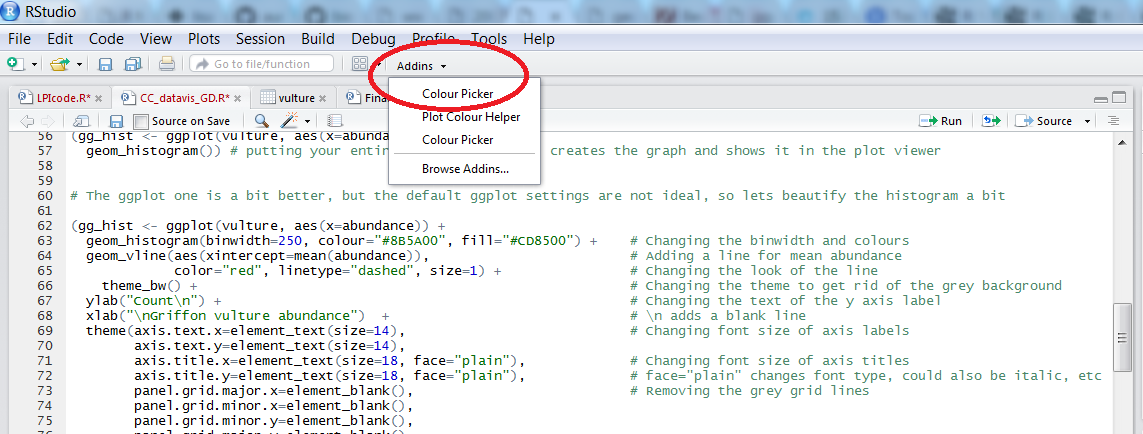When you click on `All R colours` you will see lots of different colours you can choose from - a good colour scheme makes your graph stand out, but of course, don’t go crazy with the colours. When you click on `1`, and then on a certain colour, you fill up `1` with that colour, same goes for `2`, `3` - you can add mode colours with the `+`, or delete them by clicking the bin. Once you’ve made your pick, click `Done`. You will see a line of code `c("#8B5A00", "#CD8500")` appear - in this case, we just need the colour code, so we can copy that, and delete the rest.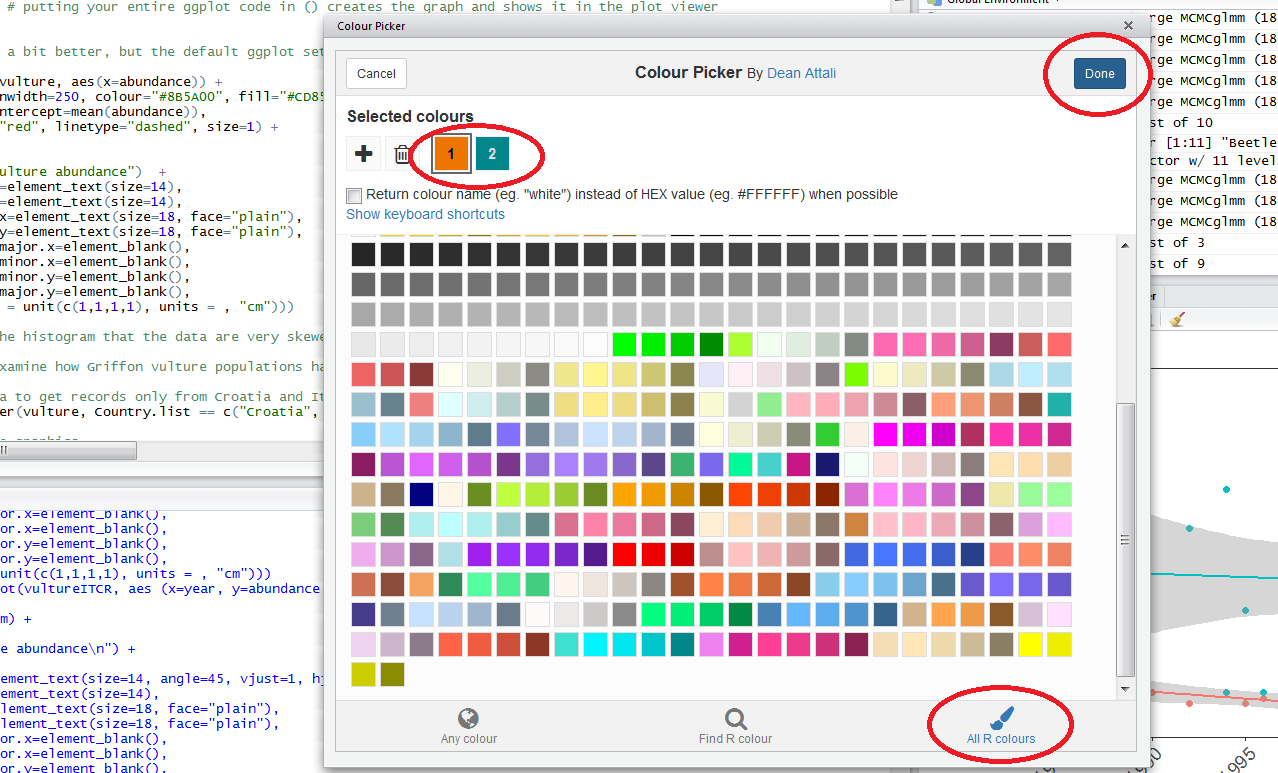## Plotting histograms of population change in different biomes and saving them

We can take our pipe efficiency a step further using the `broom` package. In the three examples above, we extracted the slope, standard error, intercept, etc., line by line, but with `broom` we can extract model coefficients using one single line `summarise(tidy(model_name)`. We can practice using `broom` whilst making the histograms of population change in different biomes (measured by the slope for the `year` term).

You will need to create a `Biome_LPI` folder, where your plots will be saved, before you run the code. This code may take a while to run, depending on your laptop. If the PDFs are taking a long time to process (e.g. `1% ~2 h remaining`), feel free press ‘Stop’ on your console and leave this code until later.

``````biome.plots <- LPI_long %>%
nest_by(genus_species_id, biome) %>% # Group by genus species ID and biome
mutate(mod =list(lm(scalepop ~ year, data = data))) %>% # Run your linear model
summarise(tidy(mod)) %>%  # Extract model coefficients
dplyr::select(genus_species_id, biome, term, estimate) %>%  # Selecting only the columns we need
spread(term, estimate)  %>% # Splitting the estimate values in two columns - one for intercept, one for year
unnest(cols = c(genus_species_id,biome)) %>% # We need to get out of our previous grouping to make a new one
do(ggsave(ggplot(., aes(x = year)) + geom_histogram(colour="#8B5A00", fill="#CD8500") + theme_LPI()
+ xlab("Rate of population change (slopes)"),
filename = gsub("", "", paste("Biome_LPI/", unique(as.character(.\$biome)), ".pdf", sep = "")), device = "pdf"))

``````

The histograms will be saved in your working directory. You can use `getwd()` to find out where that is, if you’ve forgotten. Check out the histograms - how does population change vary between the different biomes?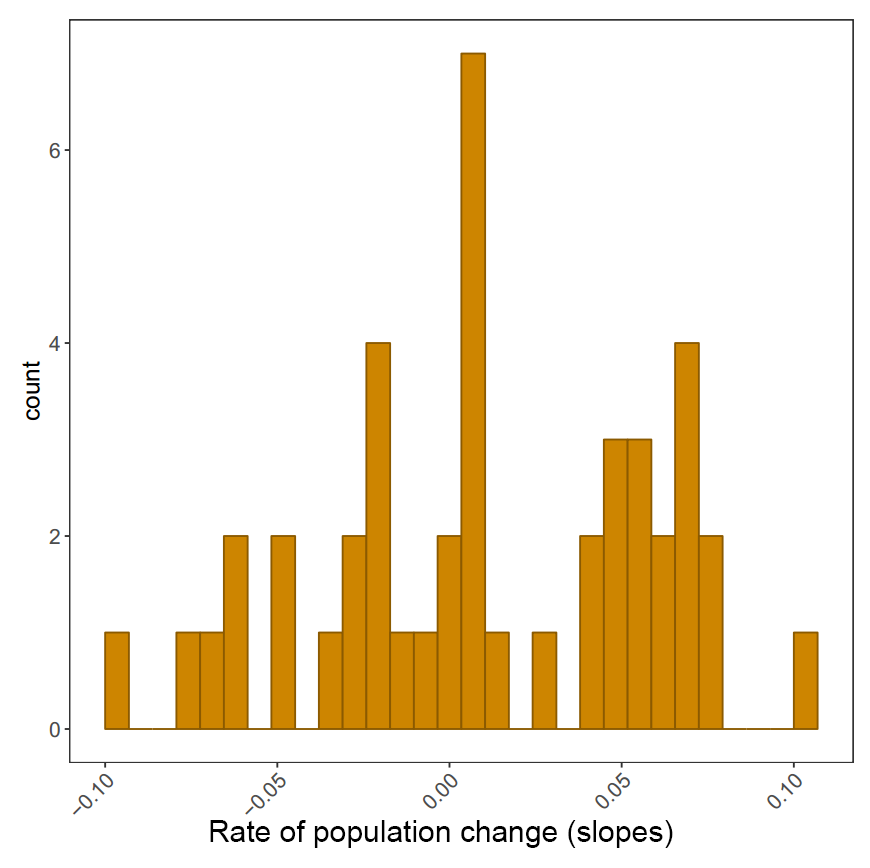## Plotting slope estimates for population change versus duration of monitoring and adding histograms along the margins

Within RStudio, you can use addins, including `Rcolourpicker` that we discussed above, and `ggExtra` that we will use for our marginal histograms.

Making our initial graph:

``````(all_slopes <- ggplot(LPI_models_pipes, aes(x = lengthyear, y = slope)) +
geom_pointrange(aes(ymin = slope - slope_se, ymax = slope + slope_se)) +
geom_hline(yintercept = 0, linetype = "dashed") +
theme_LPI() +
ylab("Population change\n") +  # \n adds a blank line
xlab("\nDuration (years)"))
``````

Note that putting your entire ggplot code in brackets () creates the graph and then shows it in the plot viewer. If you don’t have the brackets, you’ve only created the object, but haven’t visualized it. You would then have to call the object such that it will be displayed by just typing `all_slopes` after you’ve created the “all_slopes” object.

Once you’ve installed the package by running `install.packages("ggExtra")`, you can select the `ggplot2` code, click on `ggplot2 Marginal Histograms` from the Addin menu and build your plot. Once you click `Done`, the code will be automatically added to your script.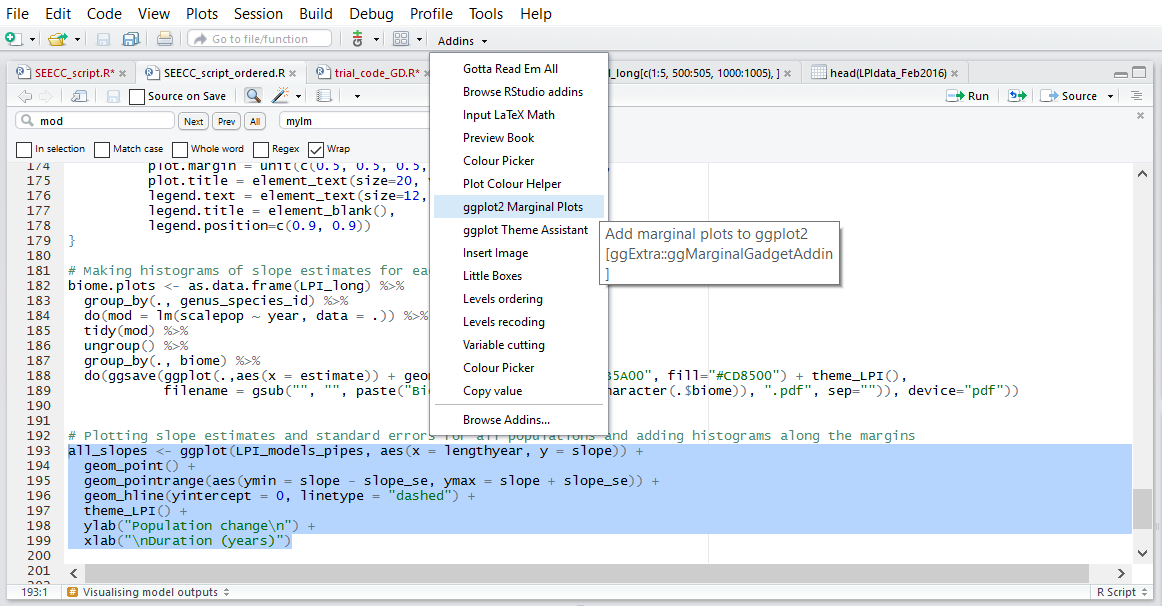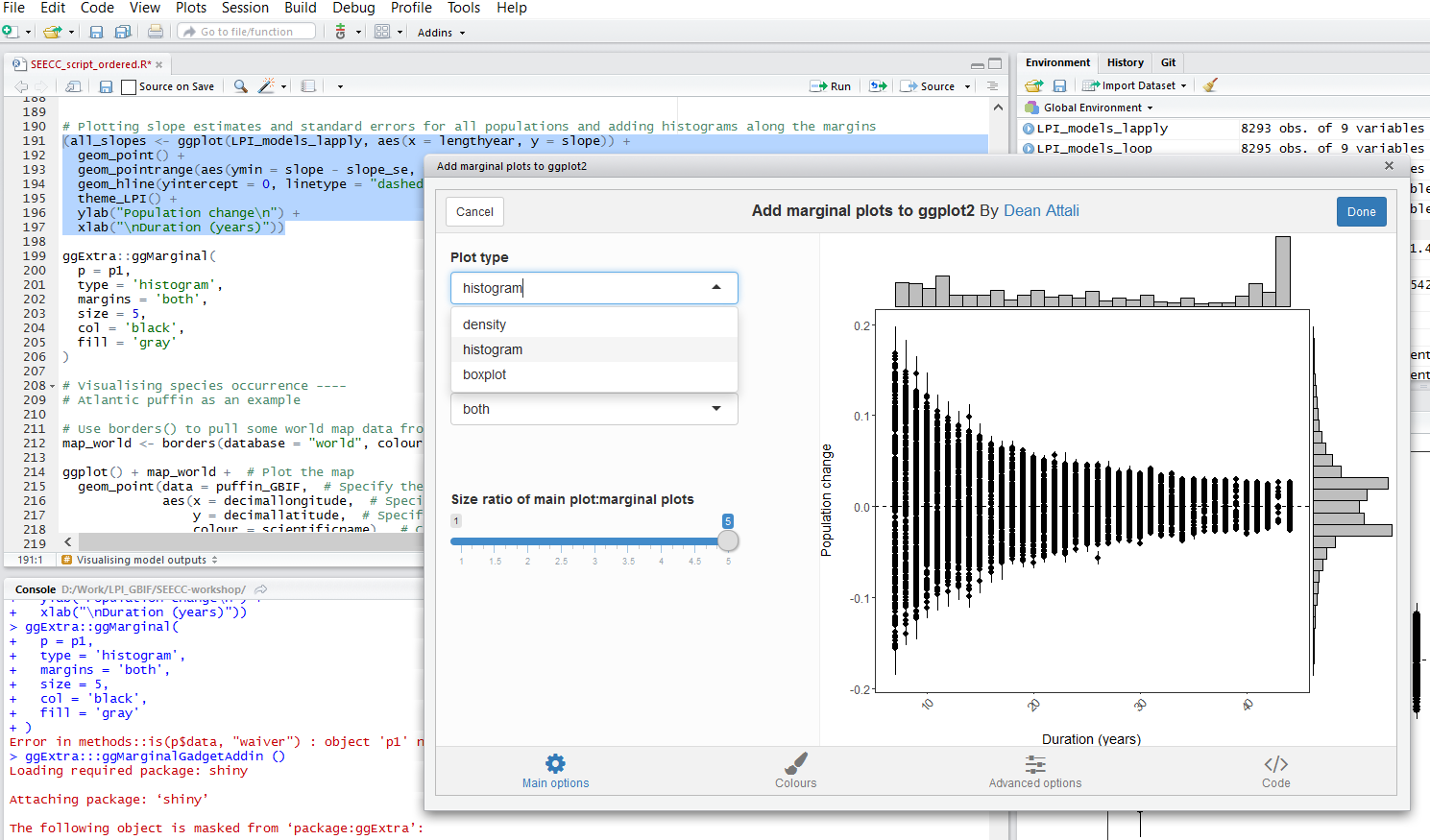Here is the final graph - what do you think, how has biodiversity changed in the last ~40 years?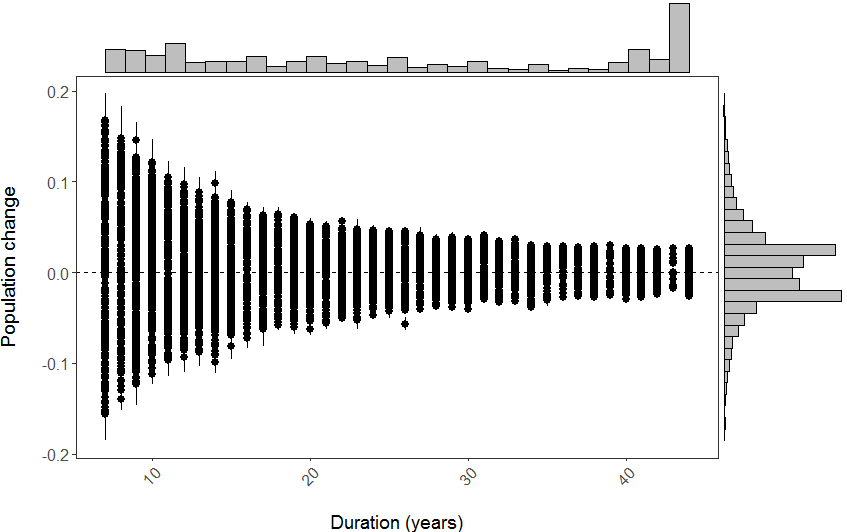## Visualising species occurrence

As an intro to the next section of the workshop, we’re going to have a look at the distribution of the Atlantic Puffin, using public data from the Global Biodiversity Information Facility, found in `puffin_GBIF.RData`.

Firstly, use `borders()` to pull some world map data from the `maps` package:

``````map_world <- borders(database = "world", colour = "gray50", fill = "#383838")  # We used the `Colour Picker` Addin to pick the colours
``````

Then create the plot using `ggplot()`:

``````(map_world_puffin <- ggplot() + map_world +  # Plot the map
geom_point(data = puffin_GBIF,  # Specify the data for geom_point()
aes(x = decimallongitude,  # Specify the x axis as longitude
y = decimallatitude,  # Specify the y axis as latitude
colour = scientificname),  # Colour the points based on species name
alpha = 0.4,  # Set point opacity to 40%
size = 1) +  # Set point size to 1
scale_color_brewer(palette = "Set1") +   # Specify the colour palette to colour the points
theme_classic() +  # Remove gridlines and shading inside the plot
ylab(expression("Latitude ("*degree*")" )) +  # Add a smarter x axis label
xlab(expression("Longitude ("*degree*")" )) +  # Add a smarter y axis label
theme(legend.position = "bottom",  # Move the legend to below the plot
legend.title = element_blank()))  # Remove the legend title
``````

We used a colour palette from `RColorBrewer` to colour the points (`Set1`). You can see all the colour palettes by running `display.brewer.all()` in R.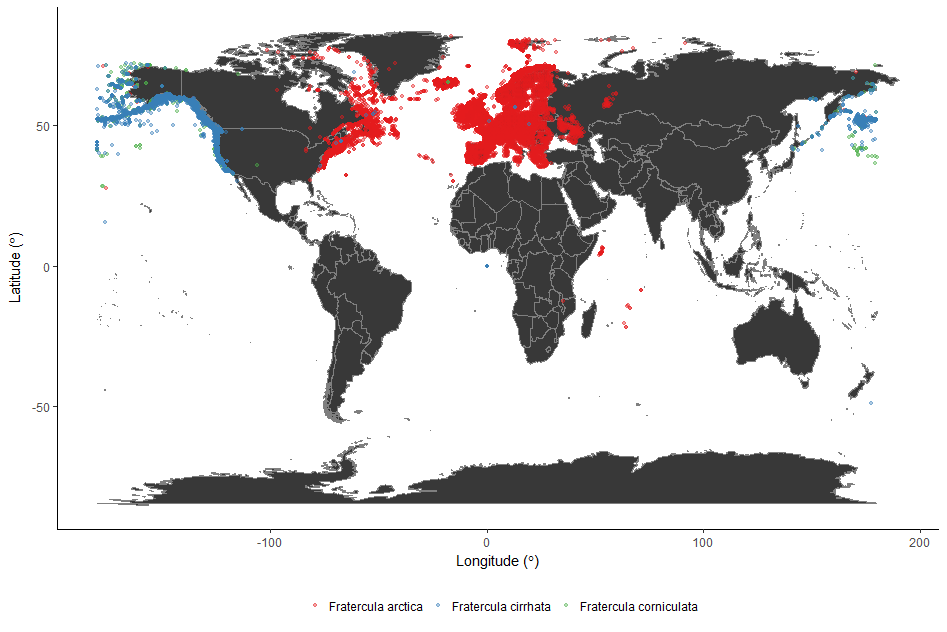# 5. Species occurrence maps based on GBIF and Flickr data

In this part of the tutorial, we will use two datasets, one from the Global Biodiversity Information Facility (GBIF) and one from Flickr, to create species occurrence maps.

So called “big data” are being increasingly used in the life sciences because they provide a lot of information on large scales and very fine resolution. However, these datasets can be quite tricky to work with. Most of the time the data is in the form of presence-only records. Volunteers, or social media users, take a picture or record the presence of a particular species and they report the time of the sighting and its location. Therefore, what we have is thousands of points with temporal and spatial information attached to them.

We will go through different steps to download, clean and visualise this type of data. We will start with downloading all the occurrences of atlantic puffin in the UK that are in the GBIF database. Then we will do some spatial manipulation of data attached to pictures of atlantic puffins taken in the UK and uploaded on Flickr. Finally, we will produce density maps of both of these datasets to look for hotspots of puffins and/or puffin watchers.

First install and load all the package needed.

``````install.packages("rgbif")
library("rgbif")
``````

The package `rgbif` offers an interface to the Web Service methods provided by GBIF. It includes functions for searching for taxonomic names, retrieving information on data providers, getting species occurrence records and getting counts of occurrence records.

In the GBIF dataset, every country has a unique code. We can find out the code for the UK with this line of code.

``````UK_code <- isocodes[grep("United Kingdom", isocodes\$name), "code"]
``````

Now, we can download all the occurrence records for the atlantic puffin in the UK using the function `occ_search`.

``````occur <- occ_search(scientificName = "Fratercula arctica", country = UK_code, hasCoordinate = TRUE, limit = 3000, year = '2006,2016', return = "data")
``````

This will return a dataset of all the occurrences of atlantic puffin recorded in the UK between 2006 and 2016 that have geographic coordinates.

Have a look at the dataset.

``````str(occur)
``````

Now we can plot the occurrences on a map of the UK. We can use ggplot to do so:

``````library(ggplot2)
library(maps)
library(ggthemes)

(map <- ggplot(occur\$data, aes(x = decimalLongitude, y = decimalLatitude)) +
# Specify to only present the UK region of the world in the map
# Also change the colour, size of map country borders
borders(database = "world", regions = "UK", colour = "gray40", size = 0.3) +
theme_map() +
# Change the colour and transparency of the plotted occurrence points
geom_point(alpha = 0.4, colour = "red"))

``````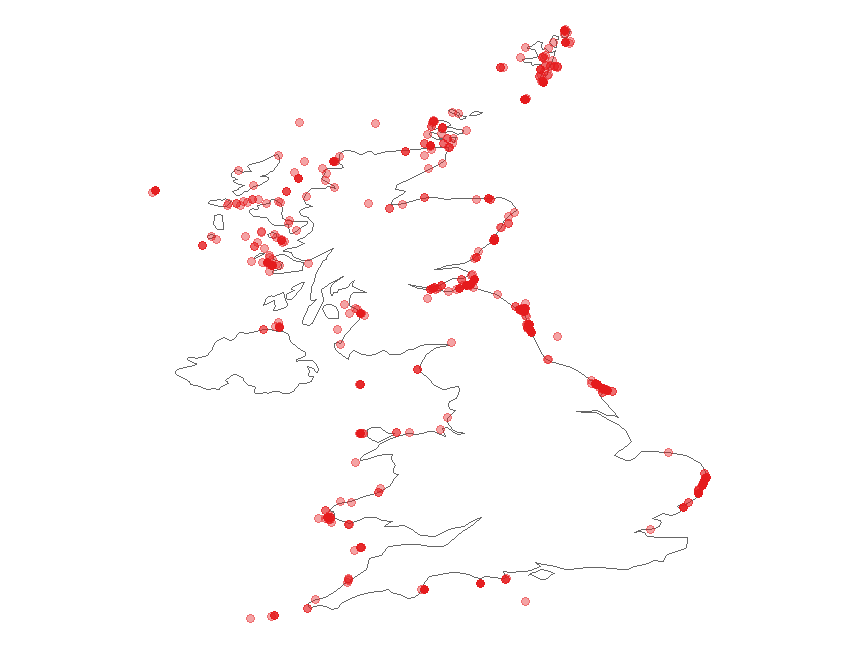## Clean data from Flickr

We will now use the dataset flickr_puffins.txt. This dataset has been collected from Flickr Application Programming Interface (API), which is an interface through which softwares can interact with each other. APIs make it easy for developers to create applications which access the features or data of an operating system, application or other service. Because of time constraints, we are not going to go through the code to download data from Flick API, but you can find the script here and I am happy to help if you find something unclear.

First, load the dataset and have a look at it.

``````flickr <- read.table("./flickr_puffins.txt", header = T, sep = "\t")
str(flickr)
``````

The variables `id` and `owner` are the unique identifier for the photograph and for the photographer, respectively. The dataset also contains the date on which the photo was taken in the original format (`datetaken`) and broken down into `month` and `year`. The variable `dateonly` is the character string containing only the date and ignoring the time when the picture was taken. The data frame also has the geographic coordinates of the place where the picture was taken.

Let’s have a first look at the data. We can quickly plot the data using the package `sp`.

``````library(sp)                                           # load the package
geopics <- flickr[, c(4,5)]                           # subset the dataset to keep coordinates only
coordinates(geopics) <- c("longitude", "latitude")    # make it spatial
plot(geopics)                                         # plot it
``````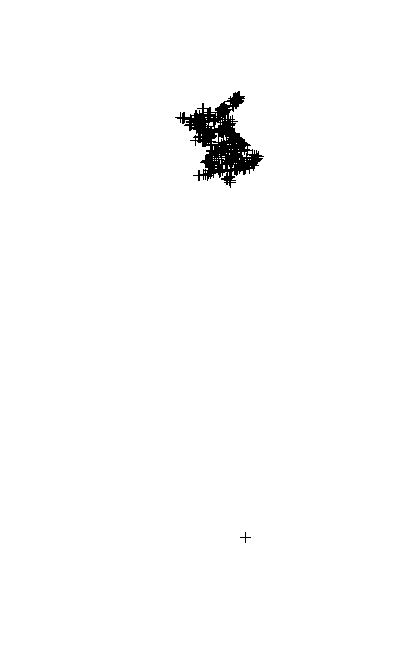The function `coordinates` sets spatial coordinates to create a Spatial object or retrieves spatial coordinates from a Spatial object.

The first thing we notice is that one point is clearly not in the UK and there are a few points in the Channel Islands, which we will delete. To do this, we just need to find which points have a latitude value that is smaller than the most southern point of the UK and delete them from the dataset.

``````which(flickr\$latitude < 49.9)
flickr <- flickr[-which(flickr\$latitude < 49.9),]
``````

Let’s check that all the points are in the UK now, using a nicer plot. We first assign a particular coordinate refernce system (CRS) to the data. A CRS defines, with the help of coordinates, how a two-dimensional map is related to real places on the earth. Since the coordinates in our dataset are recorded as decimal degrees, we can assign our spatial object a Geographic Coordinate System (GCS). WGS 1984 is the most commonly used GCS so that’s what we will use. If you want to know more about CRS in R here is a useful link.

``````coordinates(flickr) <- c("longitude", "latitude")             # go back to original dataframe and make it spatial
crs.geo <- CRS("+proj=longlat +ellps=WGS84 +datum=WGS84")     # geographical, datum WGS84
proj4string(flickr) <- crs.geo                                # assign the coordinate system
plot(flickr, pch = 20, col = "steelblue")                     # plot the data
``````

We can also add the UK coastline to the plot using the package `rworldmap`.

``````library(rworldmap)
data(countriesLow)
``````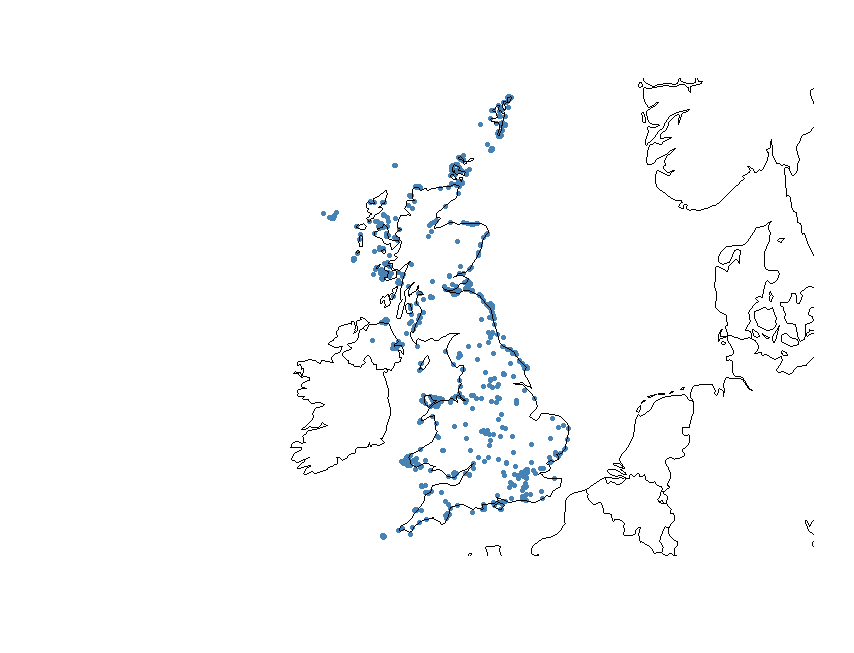There is one more problem we need to solve: some of the data points are not on the coast, which means that these pictures are probably not puffins. In order to delete them, we are going to use the UK coastline to select only the datapoints that are within 1 km of the coast and the ones that are on the sea. The first step is to split the dataset into a marine and a terrestrial one. After that, we can select only the points that are on the coast from the terrestrial dataset. Finally, we will put the marine and coastal points together. This is only one of the possible ways in which you can do this, another common one being the use of a buffer around the coastline.

First we load all the packages that we need.

``````library(rgdal)
library(rgeos)
library(raster)
library(maptools)
``````

Now we use a shapefile available in the Global Administrative Areas (GADM) database. A shapefile is a format file for storing location and attribute information of geographic features, which can be represented by points, lines or polygons.

``````UK <- getData("GADM", country = "GB", level = 0)
``````

At the moment, we are using a GCS, which means that the units of the geometry are in decimal degrees. If we want to select data points according to their distance in km from the coastline, we need to transform our spatial datasets into projected coordinate systems so the units of the distance will be in metres and not decimal degrees.

In R, it is pretty easy to transform a spatial object into a projected coordinate system. In fact, you only need one function.

``````UK_proj <- spTransform(UK, CRS("+proj=tmerc +lat_0=50 +lon_0=-2 +units=m"))
flickr_proj <- spTransform(flickr, CRS("+proj=tmerc +lat_0=50 +lon_0=-2 +units=m"))

``````

The UK shapefile is composed of many polygons. We can simplify it by dissolving the polygons. This will speed things up.

``````UK_diss <- gUnaryUnion(UK_proj)
``````

Now, we perform an overlay operation using the function `over`. This will identify which of our data points fall within the UK shapefile and return `NAs` when they do not. According to this result then we can divide our dataset into a marine dataset `NA` and a terrestrial one.

``````flickr_terr <- flickr_proj[which(is.na(over(flickr_proj, UK_diss, fn = NULL)) == FALSE),]
flickr_mar <- flickr_proj[which(is.na(over(flickr_proj, UK_diss, fn = NULL)) == TRUE),]
``````

Plot the two datasets to make sure it worked.

``````par(mfrow = c(1,2))
plot(flickr_terr)
plot(flickr_mar)
``````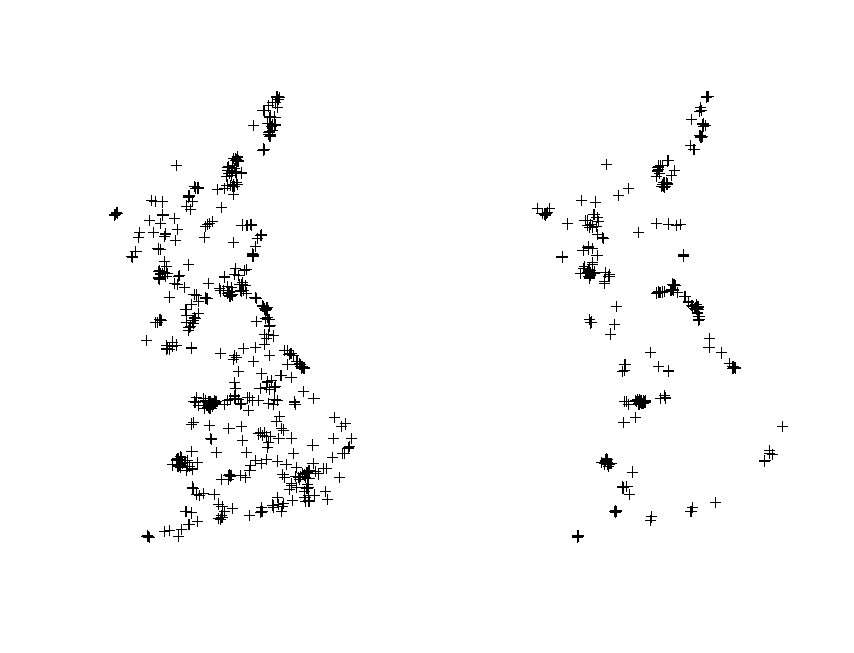Now we can select the coastal points from the terrestrial dataset. In order to calculate the distance of every point from the coastline, we need to transform our UK polygon shapefile into a line shapefile. Again, this operation is pretty straightforward in R.

``````UK_coast <- as(UK_diss, 'SpatialLines')
``````

The next chuck of code calculates the distance of every point to the coast and selects only those that are within 1 km using the function `gWithinDistance`. Then, it transforms the output into a data frame and uses it to select only the coastal points from the original Flickr dataset.

``````dist <- gWithinDistance(flickr_terr, UK_coast, dist = 1000, byid = T)
dist.df <- as.data.frame(dist)
flickr_coast <- flickr_terr[which(dist.df == "TRUE"),]
``````

Plot to check it worked.

``````plot(flickr_coast)
``````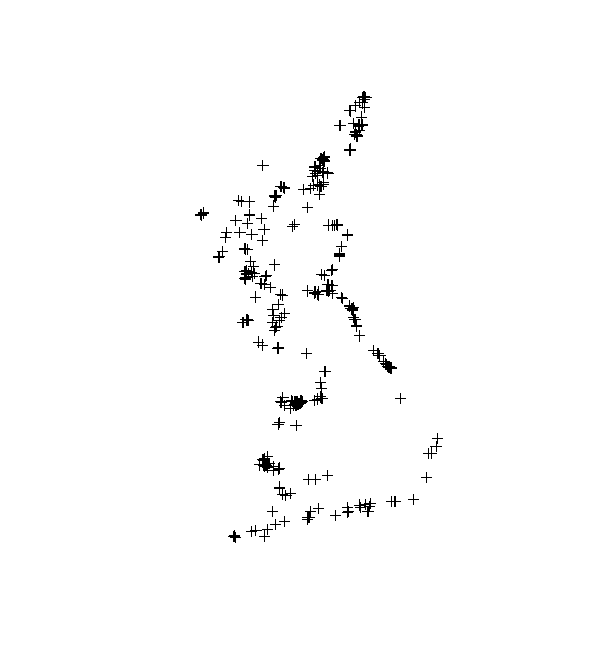Now we can put the marine and coastal datasets together and plot to check that it worked.

``````flickr_correct <- spRbind(flickr_mar, flickr_coast)
plot(UK_coast)
points(flickr_correct, pch = 20, col = "steelblue")
``````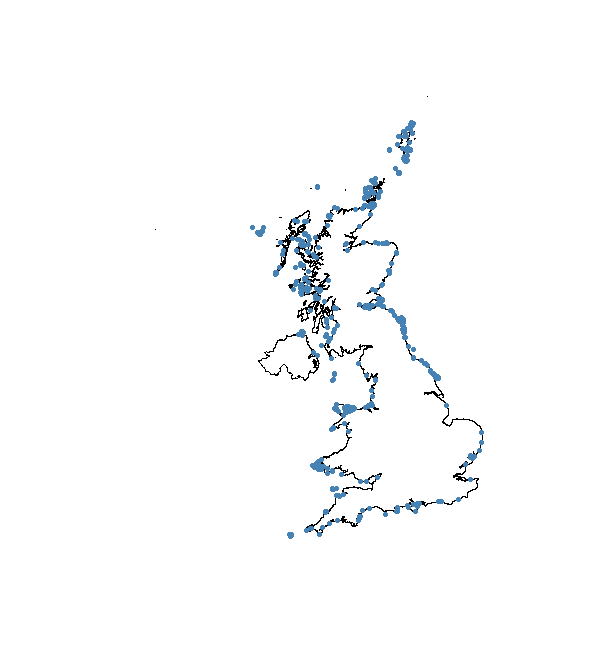## Density maps

Now that we have the datasets cleaned, it is time to make some pretty maps. When you have presence-only data, one of the things you might want to do is to check whether there are hotspots. Density maps made with `ggplot2` can help you visualise that.

We start with the Flickr data. First, we need to transform our spatial datasets into a format that `ggplot2` is able to read.

``````UK.Df <- fortify(UK_diss, region = "ID_0")
flickr.points <- fortify(cbind(flickr_correct@data, flickr_correct@coords))
``````

Now, we can build our map with `ggplot2`. If you want to know more about the way you build plots in `ggplot2` here is a useful link. One feature that you might want to take notice of is the use of `fill = ..level.., alpha = ..level..`. This syntax sets the colour and transparency of your density layer as dependent on the density itself. The `stat_` functions compute new values (in this case the `level` variable using the `kde2d` function from the package `MASS`) and create new data frames. The `..level..` tells ggplot to reference that column in the newly built data frame. The two dots indicate that the variable `level` is not present in the original data, but has been computed by the `stat_` function. This may take a while to plot, depending on your computer or laptop.

``````(plot.years <- ggplot(data = flickr.points, aes(x = longitude, y = latitude)) +  # plot the flickr data
geom_polygon(data = UK.Df,aes(x = long, y = lat, group = group),            # plot the UK
color = "black", fill = "gray82") + coord_fixed() +    # coord_fixed() ensures that one unit on the x-axis is the same length as one unit on the y-axis
geom_point(color = "dodgerblue4",size = 2,shape = ".")+                   # graphical parameters for points
stat_density2d(aes(x = longitude,                           # create the density layer based on where the points are
y = latitude,  fill = ..level.., alpha = ..level..),   # colour and transparency depend on density
geom = "polygon", colour = "grey95",size=0.3) +            # graphical parameters for the density layer
scale_fill_gradient(low = "yellow", high = "red") +                 # set colour palette for density layer
scale_alpha(range = c(.25, .5), guide = FALSE) +                    # set transparency for the density layer
facet_wrap(~ year) +                                                 # multipanel plot according to the variable "year" in the flickr dataset
theme(axis.title.x = element_blank(), axis.text.x = element_blank(),  # don't display x and y axes labels, titles and tickmarks
axis.ticks.x = element_blank(),axis.title.y = element_blank(),
axis.text.y = element_blank(), axis.ticks.y = element_blank(),
text = element_text(size = 18),legend.position = c(.9, .15),       # size of text and position of the legend
panel.grid.major = element_blank(),                            # eliminates grid lines from background
panel.background = element_blank()))                            # set white background

# This will take a while to plot!
``````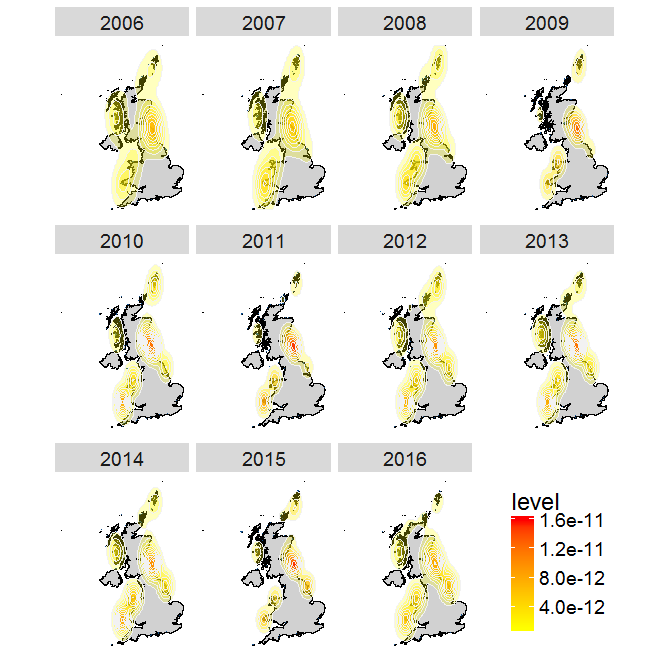You can see from this plot that there are a few hotspots for watching puffins in the UK, such as the Farne Islands, Shetland and Flamborough Head.

Now try to build your own plot for the GBIF data. Remeber to:

• make the `occur` dataset spatial with `coordinates()`
• assign the right coordinate system with `proj4string()`
• transform the coordinates to UTM with `spTransform()`
• use `fortify()` to make it readable by `ggplot2`
• build your plot with `ggplot`

If you get stuck you can find the code in the script SEECC_script_final.R here.

This tutorial was prepared for a workshop on quantifying biodiversity change at the Scottish Ecology, Environment and Conservation Conference on 3rd April in Aberdeen. If you want to learn more about our joint workshop with the Aberdeen Study Group, led by Francesca Mancini, you can check out our blog posts on the Team Shrub blog and Francesca’s blog. The workshop organisation and preparation of teaching materials were supported by the Global Environment & Society Academy Innovation Fund.